# Manual

## Introduction

The package varycoef contains methods to model and estimate varying coefficients. In its current version 0.3.1 it supports:

• only spatially varying coefficient (SVC)

• different MLE approaches to model SVC and to give predictions.

The methodology is based on (Dambon, Sigrist, and Furrer 2021).

### Spatially Varying Coefficient Models

We define a full SVC model as

$y(s) = x^{(1)}(s)\beta_1(s) + ... + x^{(p)}(s)\beta_p(s) + \epsilon(s)$

with the coefficients represented by Gaussian random fields (GRF) $$\beta_j(\cdot) \sim \mathcal N (\mu_j \textbf 1_n, C_j(\cdot, \cdot))$$. That is, every coefficient $$j = 1, ..., p$$ is distinctly defined by a mean $$\mu_j$$ and a covariance matrix defined by an underlying covariance function $$C_j(s_k, s_l) = \sigma_j^2 \phi_{\rho_j}(s_k, s_l)$$, where $$\sigma_j^2$$ is the variance and $$\rho_j$$ is the scale of the GRF. Further, $$\epsilon$$ is a nugget effect with variance $$\tau^2$$.

However, there are some cases, where the assumption of a full SVC model is not applicable. We want to give options for covariates w.r.t.:

1. mixed SVC, i.e. as above with its respective mean (fixed effect) and random effect described by its GRF.
2. fixed effect, i.e. without an GRF modelling its spatial structure.
3. mean-zero SVC, i.e. only a zero-mean random effect modelling the spatial structure.

That is why we generalize the model above. First, note that we can write it in matrix form as

$\textbf y(\textbf s) = \textbf X \beta(\textbf s) + \epsilon( \textbf s)$

where $$\textbf X$$ is our model matrix. Then we can write down the model divided into an fixed effect part and a random effect part:

$\textbf y(\textbf s) = \textbf X \mu + \textbf W \eta(\textbf s) + \epsilon( \textbf s)$

where $$\eta$$ is the joint mean-zero GRF. Note that both model are the same if $$\textbf X = \textbf W$$. Thus, we can specify options 1 to 3 from above by in- or excluding columns of $$\textbf X$$ or $$\textbf W$$, respectively.

### Example

To give a simple example, we start by sampling artificial data. So define an full SVC model as given above and sample from a regular grid using a help function:

# number of SVC
p <- 3

(pars <- data.frame(mean = rep(0, p),
var = c(0.1, 0.2, 0.3),
scale = c(0.3, 0.1, 0.2)))
##   mean var scale
## 1    0 0.1   0.3
## 2    0 0.2   0.1
## 3    0 0.3   0.2
nugget.var <- 0.05

# sqrt of total number of observations
m <- 10; n <- m^2
locs <- expand.grid(
x = seq(0, 1, length.out = m),
y = seq(0, 1, length.out = m)
)

# function to sample SVCs
sp.SVC <- sample_fullSVC(
df.pars = pars,
nugget.sd = sqrt(nugget.var),
locs = as.matrix(locs),
cov.name = "exp"
)
str(sp.SVC)
## List of 5
##  $y : num [1:100] 0.0891 0.1982 0.1161 0.9915 -0.3224 ... ##$ X   : num [1:100, 1:3] 1 1 1 1 1 1 1 1 1 1 ...
##  $beta: num [1:100, 1:3] 0.2726 0.4663 0.2118 0.5003 -0.0107 ... ##$ eps : num [1:100] -0.41051 -0.12661 0.00156 0.11458 -0.21981 ...
##  $locs: num [1:100, 1:2] 0 0.111 0.222 0.333 0.444 ... ## ..- attr(*, "dimnames")=List of 2 ## .. ..$ : NULL
##   .. ..$: chr [1:2] "x" "y" head(cbind(y = sp.SVC$y, X = sp.SVC$X)) ## y ## [1,] 0.08910103 1 1.7743311 -0.6309346 ## [2,] 0.19823586 1 0.2458067 0.7198744 ## [3,] 0.11612723 1 0.4317260 1.0392399 ## [4,] 0.99154869 1 -0.5105951 -0.6360565 ## [5,] -0.32241395 1 0.5798840 0.2447040 ## [6,] -0.91198405 1 -1.4306570 1.1137884 ## MLE in varycoef The main function of this package is SVC_mle. Its function call starts the MLE but it requires some preparation and settings of control parameters. We go through each argument of the SVC_mle function and its control parameter SVC_mle.control. ### The Function SVC_mle As one might see in the help file of the SVC_mle function, it has 3 mandatory arguments: y, the response; X, the data matrix and locs, the locations. If we do not change W, i.e. W = NULL, then we use W = X and are in the case of a full SVC. We will give examples for different kinds of models. ### Control Parameters As for the control parameters for SVC_mle, we go through them as they are implemented in the current version of varycoef. By calling SVC_mle_control, we create an list with all needed arguments to start a simple SVC_mle. control <- SVC_mle_control() str(control) ## List of 13 ##$ cov.name   : chr "exp"
##  $tapering : NULL ##$ parallel   : NULL
##  $init : NULL ##$ lower      : NULL
##  $upper : NULL ##$ save.fitted: logi TRUE
##  $profileLik : logi FALSE ##$ mean.est   : chr "GLS"
##  $pc.prior : NULL ##$ extract_fun: logi FALSE
##  $hessian : logi TRUE ##$ dist       :List of 1
##   ..$method: chr "euclidean" #### Covariance Function Here we define the covariance function $$C_j(s_k, s_l) = \sigma_j^2 \phi_{\rho_j}(s_k, s_l)$$. In its current version 0.3.1, varycoef supports only exponential covariance functions, i.e. $$\phi_{\rho}(s_k, s_l) = \exp\left(\frac{\|s_k - s_l\|}{\rho}\right)$$. #### Tapering Covariance tapering goes back to (Furrer, Genton, and Nychka 2006) and is a technique to challenge the “big $$n$$ problem” when dealing with spatial data. When working with $$n$$ observations, the covariance matrix has dimension $$n \times n$$. The likelihood function of a single GRF or, in particular, the SVC models as described above, includes the inverse as well as the determinant of a covariance matrix. The computation of both is based on the Cholesky decomposition which has run-time $$\mathcal O(n^3)$$. There are several ways on how to deal with this computational burden. With covariance tapering, we introduce a sparsity structure on the covariance matrix. This sparsity structure can then be used to our advantage with the Cholesky decomposition implemented in the package spam, (Furrer and Sain 2010). In a nutshell, this decomposition becomes faster as the sparsity increases. However, this comes with a trade-off in the precision of the MLE of the covariance parameters with finitely many observation. Asymptotically, the procedure is consistent. By default, the tapering entry is NULL, i.e. no tapering is applied. If a scalar is provided, then this is taper range is applies. In other words, every spatial dependency is cut for distances larger than tapering. We illustrate the difference between both the untapered and tapered covariance matrix of the SVC on the regular grid example from above. The function fullSVC_reggrid is used to sample SVCs for a full SVC model.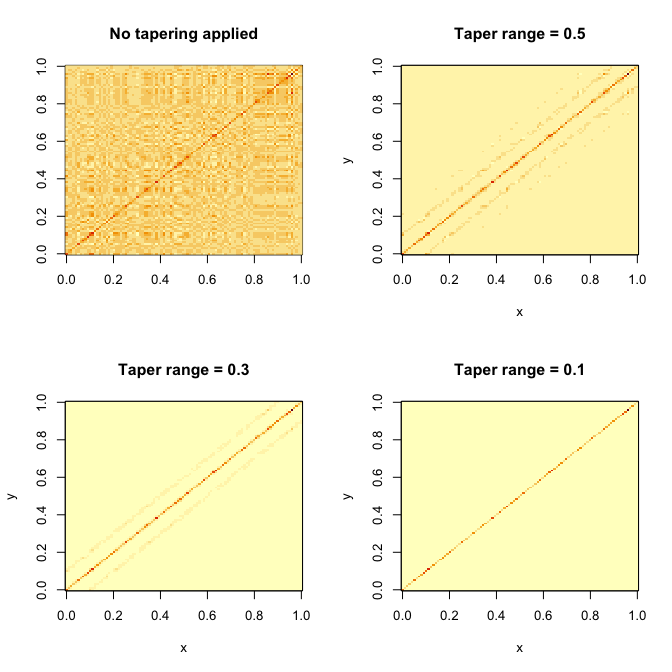Finally, we show the time differences of evaluating the likelihood function between the different taper ranges. We use the microbenchmark package: library(microbenchmark) (mb <- microbenchmark(no_tapering = out[][](), tapering_0.5 = out[][](), tapering_0.3 = out[][](), tapering_0.1 = out[][]()) ) ## Unit: milliseconds ## expr min lq mean median uq max neval ## no_tapering 3.327043 3.732810 4.761137 3.951540 4.581335 14.549345 100 ## tapering_0.5 3.673749 4.181364 5.608183 4.829471 5.591309 23.287474 100 ## tapering_0.3 2.726926 2.985486 3.659342 3.381055 3.911504 13.108583 100 ## tapering_0.1 2.116330 2.298726 2.808169 2.564931 2.957707 7.579552 100 boxplot(mb, unit = "ms", log = TRUE, xlab = "tapering", ylab = "time (milliseconds)")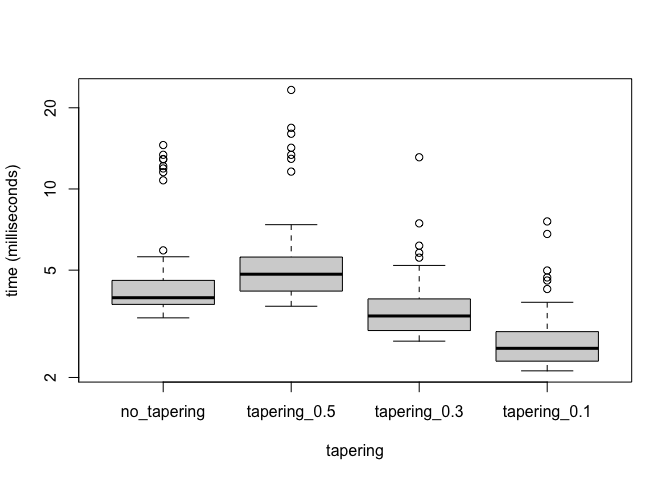#### Regularizing Priors We implemented a usage of penalizing complexity priors. The argument pc.prior takes a vector of length 4 as an input where the values are $$\rho_0, \alpha_\rho, \sigma_0, \alpha_\sigma$$ to compute penalized complexity priors. One wants to penalize the lower tail on the range parameter as well as the upper tail of the standard deviation: $P(\rho < \rho_0) = \alpha_\rho, \quad P(\sigma > \sigma_0) = \alpha_\sigma.$ #### Parallelization With version 0.2.10 varycoef is now able to parallelize the likelihood optimization. In each iteration step the objective function, i.e., a modified likelihood, has to be evaluated at several points in a small neighborhood. Using the package optimParallel (Gerber and Furrer 2019), is can be done simultaneously. The procedure to do so is the following: 1. Initialize a cluster by parallel::makeCluster. 2. Create list containing this cluster, as one would with optimParallel::optimParallel. In this list other arguments towards the function optimParallel can be passed, see help file. 3. Set argument parallel to the created list. 4. Run SVC_mle as usual. 5. Make sure to stop cluster afterwards. The code looks something like that: require(varycoef) require(parallel) require(optimParallel) # step 1: initialize cluster cl <- makeCluster(detectCores()-1) # step 2: create optimParallel control parallel.control <- list(cl = cl, forward = TRUE, loginfo = FALSE) # step 3: add control containing optimParallel controls control.p <- control control.p$parallel <- parallel.control

# step 4: run SVC_mle
fit.p <- SVC_mle(y = y, X = X, locs = coordinates(sp.SVC),
control = control.p)

# step 5: stop cluster
stopCluster(cl); rm(cl)

summary(fit.p)
rm(control.p, fit.p)

#### Extract Function

In some situations, it is useful to extract the objective function before starting the optimization itself. For instance, (Gerber and Furrer 2019) states that the overhead of the parallelization set up results in a faster optimization only if the evaluation time of a single objective function is greater than 0.05 seconds. Another example where the extracted function is needed are machine specific issues regarding the optimization.

### Example: MLE of full SVC

We can now start the MLE . The following function call takes a few second.

control <- SVC_mle_control()
fit <- SVC_mle(y = sp.SVC$y, X = sp.SVC$X, locs = as.matrix(locs), control = control)

The received object fit is of class SVC_mle. For this class, there are numerous methods such as:

# estimated ...
# ... covariance parameters
cov_par(fit)
## SVC1.range   SVC1.var SVC2.range   SVC2.var SVC3.range   SVC3.var nugget.var
## 0.06502286 0.08602431 0.11557192 0.19400785 0.18390710 0.40452953 0.00000100
## attr(,"cov_fun")
##  "exp"
 # ... mean effects
coef(fit)
##          Var1          Var2          Var3
##  0.0219403586 -0.0002346972 -0.2013061550
# summary
summary(fit)
##
## Call:
## SVC_mle.default(y = sp.SVC$y, X = sp.SVC$X, locs = as.matrix(locs),
##     control = control)
##
## Fitting a GP-based SVC model with 3 fixed effect(s) and 3 SVC(s)
## using 100 observations at 100 different locations / coordinates.
##
## Residuals:
##       Min.     1st Qu.      Median     3rd Qu.        Max.
## -6.922e-06  -1.003e-06   2.855e-08   8.658e-07   6.828e-06
##
## Residual standard error: 1.923e-06
## Multiple R-squared:     1, BIC: 216.7
##
##
## Coefficients of fixed effect(s):
##        Estimate Std. Error Z value Pr(>|Z|)
## Var1  0.0219404  0.0645820   0.340    0.734
## Var2 -0.0002347  0.1151208  -0.002    0.998
## Var3 -0.2013062  0.2208106  -0.912    0.362
##
##
## Covariance parameters of the SVC(s):
##            Estimate Std. Error W value  Pr(>W)
## SVC1.range 0.065023   0.150203      NA      NA
## SVC1.var   0.086024   0.379062   0.052 0.82047
## SVC2.range 0.115572   0.055289      NA      NA
## SVC2.var   0.194008   0.065173   8.862 0.00291 **
## SVC3.range 0.183907   0.092147      NA      NA
## SVC3.var   0.404530   0.160699   6.337 0.01183 *
## nugget.var 0.000001   0.381065      NA      NA
## ---
## Signif. codes:  0 '***' 0.001 '**' 0.01 '*' 0.05 '.' 0.1 ' ' 1
##
## The covariance parameters were estimated using
## exponential covariance functions.
## No covariance tapering applied.
##
##
## MLE:
## The MLE terminated after 43 function evaluations with convergence code 0
## (0 meaning that the optimization was succesful).
## The final log likelihood value is -94.52.
# residual plots
oldpar <- par(mfrow = c(1, 2))
plot(fit, which = 1:2)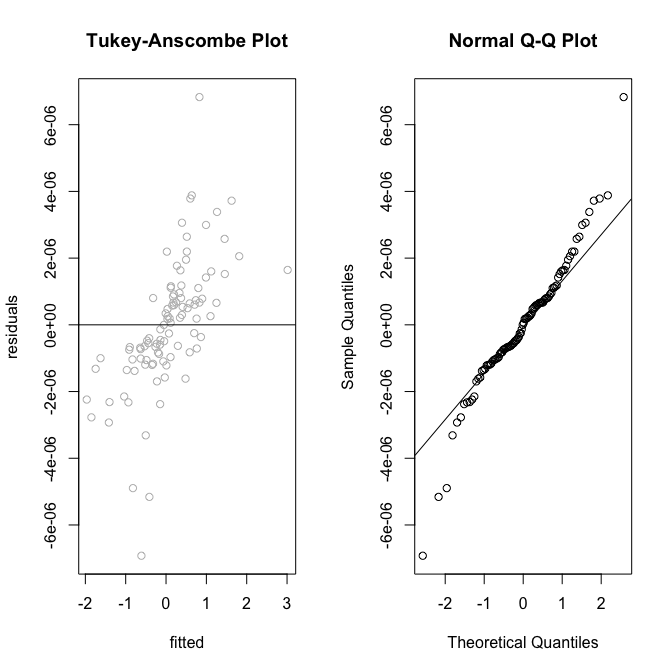par(mfrow = c(1, 1))

par(oldpar)

Now, we can use our fit object to make predictions:

# calling predictions without specifying new locations (newlocs) or
# new covariates (newX) gives estimates of SVC only at the training locations.
pred.SVC <- predict(fit)

Since we know the true SVC, we can compute the error in prediction and compare it to the true values.

library(sp)
colnames(pred.SVC)[1:p] <- paste0("pred.",colnames(pred.SVC)[1:p])
coordinates(pred.SVC) <- ~loc_1+loc_2
all.SVC <- cbind(pred.SVC, sp.SVC$beta) # compute errors all.SVC$err.SVC_1 <- all.SVC$pred.SVC_1 - all.SVC$X1
all.SVC$err.SVC_2 <- all.SVC$pred.SVC_2 - all.SVC$X2 all.SVC$err.SVC_3 <- all.SVC$pred.SVC_3 - all.SVC$X3

colnames(all.SVC@data) <- paste0(rep(c("pred.", "true.", "err."), each = p), "SVC_", rep(1:p, 3))

spplot(all.SVC[, paste0(rep(c("true.", "err.", "pred."), each = p),
"SVC_", 1:p)], colorkey = TRUE)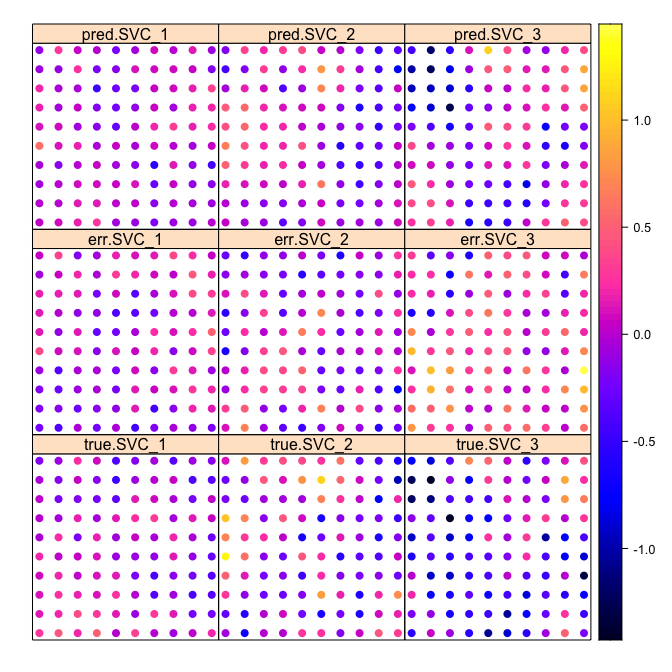In this small example we already can see that the predicted SVC takes the general spatial structure of the true SVC. The error does not appear to have spatial structure for the SVC 2 and 3, respectively. However, the error for the intercept seems to have some spatial structure. If we increase the number of observations, the picture changes:

knitr::include_graphics("figures/SVCs_result_n2500_p3.png")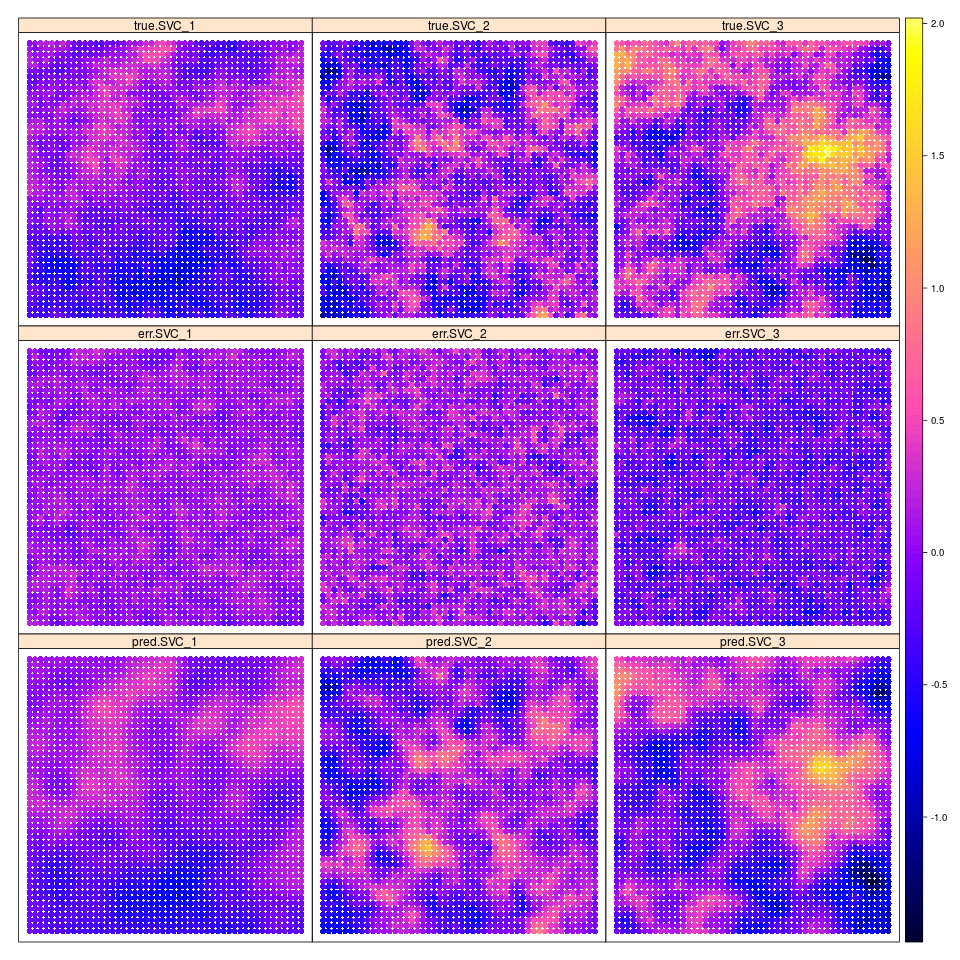We do not run the code since it takes a couple hours to do the MLE without parallelization, but here is the code to reproduce the figure:

# new m
m <- 50

# new SVC model
sp.SVC <- fullSVC_reggrid(m = m, p = p,
cov_pars = pars,
nugget = nugget.var)

spplot(sp.SVC, colorkey = TRUE)

# total number of observations
n <- m^2
X <- matrix(c(rep(1, n), rnorm((p-1)*n)), ncol = p)
y <- apply(X * as.matrix(sp.SVC@data[, 1:p]), 1, sum) + sp.SVC@data[, p+1]

fit <- SVC_mle(y = y, X = X, locs = coordinates(sp.SVC))

sp2500 <- predict(fit)

colnames(sp2500)[1:p] <- paste0("pred.",colnames(sp2500)[1:p])
coordinates(sp2500) <- ~loc_x+loc_y
all.SVC <- cbind(sp2500, sp.SVC[, 1:3])

# compute errors
all.SVC$err.SVC_1 <- all.SVC$pred.SVC_1 - all.SVC$SVC_1 all.SVC$err.SVC_2 <- all.SVC$pred.SVC_2 - all.SVC$SVC_2
all.SVC$err.SVC_3 <- all.SVC$pred.SVC_3 - all.SVC\$SVC_3

colnames(all.SVC@data) <- paste0(rep(c("pred.", "true.", "err."), each = p), "SVC_", rep(1:p, 3))

png(filename = "figures/SVCs_result_n2500_p3.png", width = 960, height = 960)
spplot(all.SVC[, paste0(rep(c("true.", "err.", "pred."), each = p),
"SVC_", 1:p)], colorkey = TRUE,
as.table = TRUE, layout = c(3, 3))
dev.off()